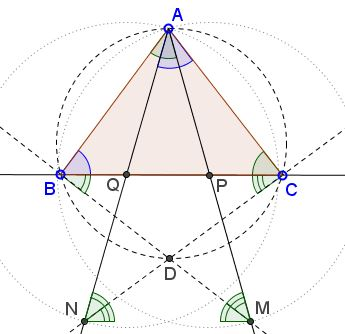# Concyclic Points at the IMO 2014

### Problem 4, IMO 2014

Let $P$ and $Q$ be on segment $BC$ of an acute triangle $ABC$ such that $\angle PAB=\angle BCA$ and $\angle CAQ=\angle ABC$. Let $M$ and $N$ be the points on $AP$ and $AQ$, respectively, such that $P$ is the midpoint of $AM$ and $Q$ is the midpoint of $AN$.Prove that the intersection $D$ of $BM$ and $CN$ is on the circumference of triangle $ABC$.

### Complex Numbers

We shall treat points in the plane as complex numbers and use the same notation for both.

Triangles $ABC$ and $PBA$ are similar, with different orientations, implying

(1)

$\displaystyle\frac{A-P}{B-P}=\bigg(\frac{C-A}{B-A}\bigg)^{*}.$

Where the asterisk denotes complex conjugation. Similarly, $\Delta ACB\sim\Delta QCA$ so that

(2)

$\displaystyle\frac{C-Q}{A-Q}=\bigg(\frac{C-A}{B-A}\bigg)^{*}.$

But

(3)

\begin{align} M-P&=-(A-P),\\ N-Q&=-(A-Q). \end{align}

Combining (1), (2), (3) gives

$\displaystyle\frac{M-P}{B-P}=\frac{C-Q}{N-Q},$

implying that triangles $MPB$ and $CQN$ are similar. Now it is obvious that $\angle ABM+\angle ACN=\pi,$ so that quadrilateral $ABDC$ is indeed cyclic.

### Analytic Geometry

Choose the Cartesian coordinates so that $A=(0,2),$ $B=(-2b,0),$ $C=(2c,0).$ Then $b=\cot B$ and $c=\cot C.$ From these,

$\displaystyle\cot A=-\cot(B+C)=\frac{1-bc}{b+c}.$

Let $P=(2p,0),$ with $p\in(-b,c).$

In $\Delta APB,$ $\angle APB=A$ and $p=\cot\angle APB,$ implying $\displaystyle p=\frac{1-bc}{b+c},$ which delivers $\displaystyle P=(2\frac{1-bc}{b+c},0).$ Similarly, $\displaystyle Q=(-2\frac{1-bc}{b+c},0).$ By the construction, $\displaystyle P=(4\frac{1-bc}{b+c},-2)$ and $\displaystyle N=(-4\frac{1-bc}{b+c},-2).$

The equation of $BM$ is $\displaystyle x=-2b-\bigg(b+2\frac{1-bc}{b+c}\bigg)y;\,$ that of the circumcircle $(ABC)$ is

$[x=(c-b)]^{2}+[y-(1-bc)]^{2}=(1+b^{2})(1+c^{2}).$

By straightforward calculations, the second intersection of the two is

$\displaystyle D=\bigg(2\frac{(c-b)(2-bc)}{(c-b)^{2}+4},-2\frac{(c+b)^{2}}{(c-b)^{2}+4}\bigg).$

Since this expression is symmetric in $b$ and $c,$ $D$ is also the intersection of $CQ$ with the circumcircle and so $D=BM\cap CQ$ and lies on the circle.

### Euclidean Geometry

This could be considered as a synthetic interpretation of the solution in complex numbersBy simple angle chasing, $\angle APB=\angle AQC=\angle BAC\,$ such that triangles $ABP$ and $CAQ$ have the same angles and are, therefore, similar:

$\displaystyle\frac{BP}{AP}=\frac{CQ}{AQ}.$

By the construction, $MP=AP$ and $NQ=AQ$ so that also

$\displaystyle\frac{BP}{MP}=\frac{CQ}{NQ}.$

Since $\angle BPM=180^{\circ}-\angle APB$ and $\angle NQC=180^{\circ}-\angle AQC,$ we also have $\angle BPM= \angle NQC,$ making triangles $BPM$ and $NQC$ similar. Now, say

\begin{align} \angle BAC = \angle AQP &= \angle ANM\\ &=\angle QNC+\angle MNC\\ &=\angle MBC+\angle NCB\\ &=\angle DBC+\angle DCB\\ &=180^{\circ}-\angle BDC, \end{align}

so that $\angle BAC+\angle BDC=\pi,\,$ and the quadrilateral $ABDC$ is indeed cyclic.

Note: $D$ lies on the circumcircle of $\Delta ABC\,$ regardless whether it is acute or not. It does not appear that this condition may affect the solution in any way.

### Acknowledgment

The solutions in complex numbers and analytic geometry are due to Leo Giugiuc (Romania).

### What Can Be Multiplied?